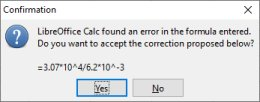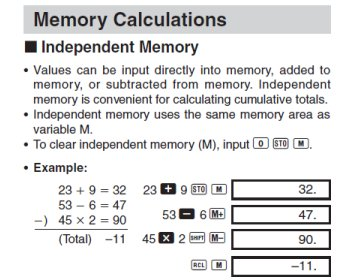Contents...

 >

 <

 Maths... Lesson 1: EXP
 I found myself on a video about the EXP button; it took me a while to find it, but there it was right at the bottom! The tutorial was to calculate 3.07 x 104 Of course you could do 3.07 x 10000 But on the calculator I can do 3.07 [EXP] 4 Where 4 refers to the number of zeros. Both result in 30700. EXP is referred to as 'Exponent' (although I would refer to the sum as "10 to the power of 4". In my maths book's Index it has the following entries for Exponent: exponential constant exponential expression exponential decay exponential function graph exponential growth These are all covered in the section on Exponential Function. I've heard of exponential growth, but let's first look at constant and expression. The book uses the example of 40.3 = 1.516 but how to get this on my calculator? I continue with the video and learn about the +/- button on my calculator so that I can do 3.07 x 104 / 6.2x10-3 3.07 [EXP] 4 / 6.2 [+/-] 3 = 4951612.9 I then wondered how I could calculate this in LibreOffice Calc. Referring to the Help there it explains this: Scientific notation lets you write very large numbers or very small fractions in a compact form. For example, in scientific notation, 650000 is written as 6.5 x 10^5, and 0.000065 as 6.5 x 10^-5. In LibreOffice, these numbers are written as 6.5E+5 and 6.5E-5, respectively. To create a number format that displays numbers using scientific notation, enter a # or 0, and then one of the following codes E-, E+, e- or e+. So I gave it a try and threw in =3.07x10^4/6.2x10^-3 LibreOffice Calc then gave me an error!Of course I hadn't fully read the instructions so I paid more attention and came up with: =3.07E4/6.2E-3 This resulted in the correct answer of 4951612.90322581 (although with 5 more digits than the calculators could display) Now I thought I could do that sum in the book: 40.3 ... in Calc... but no... it resulted in #NAME? when I put in =4E0.3 ... back to the video... I then got onto "switching modes" on my calculator when I watched this video. I couldn't get the same results. Along of the top of my calculator, beneath the screen, it has a legend/key referring to the different modes. You're supposed to be able to press the MODE button then a number corresponding to the MODE you want; it's on 4/DEG by default. I could switch it to 5/RAD, 6/GRA, but not 7/FIX or 8/SCI. I hadn't been able to find a manual for my exact calculator (Casio fx-85VH) but I found one for the fx-85W/WA. I then got lost in this little exercise:I thought this was pretty neat even though my calculator has MR instead of RCL (as in Memory Recall, and Recall respectively). I then watched a bit more of this video and discovered that I need to do more than press MODE 8 for Scientific, I need to choose a number between 0 and 9, the video says to choose 9. Hey-presto, it displays 0.000000000 I still can't do 40.3 but in my maths book, following that sum, it talks about ex where e is the exponential constant, 2.71828 . . . . I find that button on my calculator and can do e1.3 but I still can't do 40.3 . . . .